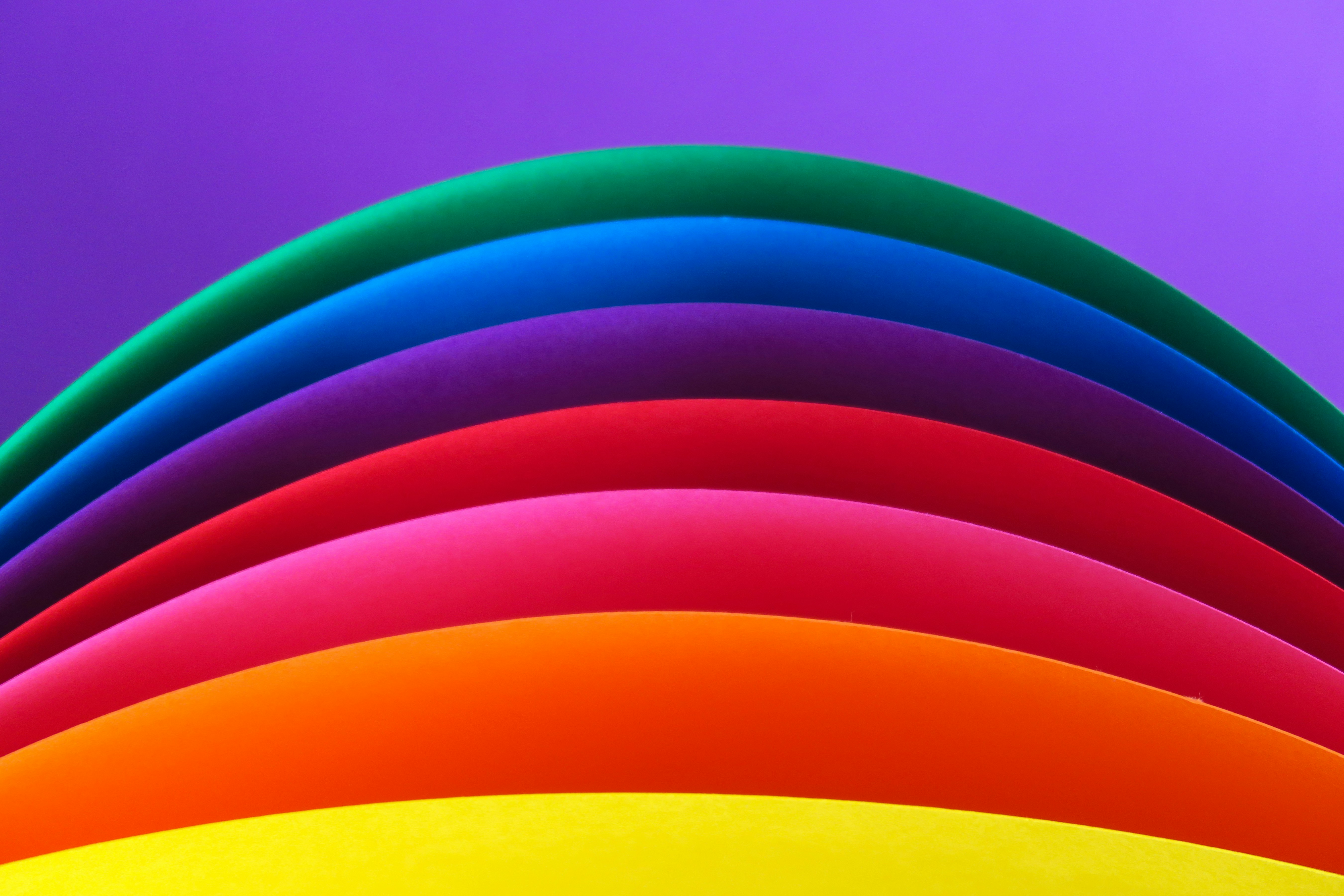# 🌈 Color Conversion with PHP From HEX to RGB and BackPhoto by Daniele Levis Pelusi / Unsplash

Following Color class will allow you to convert colors from RGB to hex and back

``````class Color
{
/** int \$red */
public \$red;

/** int \$green */
public \$green;

/** int \$blue */
public \$blue;

/**
* Color constructor.
* @param \$red
* @param \$green
* @param \$blue
*/
public function __construct(\$red, \$green, \$blue)
{
\$this->red = \$red;
\$this->green = \$green;
\$this->blue = \$blue;
}

// ...
}
``````

Convert from HEX string to RGB add this function to our class

``````public static function convertToRGB(\$hex)
{
\$hex = ltrim(\$hex, "#");

if (!ctype_xdigit(\$hex))
throw new NotHexException();

\$red = hexdec(substr(\$hex, 0, 2));
\$green = hexdec(substr(\$hex, 2, 2));
\$blue = hexdec(substr(\$hex, 4, 2));

return new Color(\$red, \$green, \$blue);
}
``````

This is how you can convert from rgb back to HEX string.

``````public static function convertToHex(Color \$color)
{
\$red = dechex(\$color->red);
if (strlen(\$red) < 2) \$red = '0' . \$red;

\$green = dechex(\$color->green);
if (strlen(\$green) < 2) \$green = '0' . \$green;

\$blue = dechex(\$color->blue);
if (strlen(\$blue) < 2) \$blue = '0' . \$blue;

return '#' . \$red . \$green . \$blue;
}
``````

One thing is missing and it's exception which is used in `convertToRGB` function:

``````class NotHexException extends \Exception
{
public function __construct(\$message = "String you have provided is not HEX", \$code = 0, Throwable \$previous = null)
{
parent::__construct(\$message, \$code, \$previous);
}
}
``````

To use it

``````use MayMeow\PHPColor\Color;
// ...
\$color = new Color(198, 255, 32);
\$hex = Color::convertToHex(\$color); //#c6ff20
``````

and back to RGB

``````use MayMeow\PHPColor\Color;
// ...
try {
\$rgb = Color::convertToRGB(\$hex); // color object: Color(198, 255, 32)
} catch (\MayMeow\PHPColor\Exceptions\NotHexException \$exception)
{
// do something, echo message or log ...
}
``````